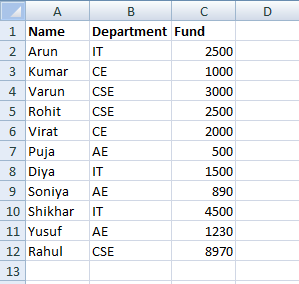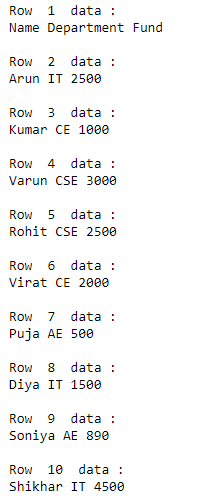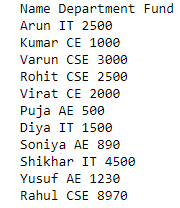GeeksforGeeks App
Open AppBrowser
Continue

# How to iterate through Excel rows in Python?

In this article, we are going to discuss how to iterate through Excel Rows in Python. In order to perform this task, we will be using the Openpyxl module in python. Openpyxl is a Python library for reading and writing Excel (with extension xlsx/xlsm/xltx/xltm) files. The openpyxl module allows a Python program to read and modify Excel files.

We will be using this excel worksheet in the below examples:Approach #1:

We will create an object of openpyxl, and then we’ll iterate through all rows from top to bottom.

## Python3

 `# import module``import` `openpyxl`` ` `# load excel with its path``wrkbk ``=` `openpyxl.load_workbook(``"Book1.xlsx"``)`` ` `sh ``=` `wrkbk.active`` ` `# iterate through excel and display data``for` `i ``in` `range``(``1``, sh.max_row``+``1``):``    ``print``(``"\n"``)``    ``print``(``"Row "``, i, ``" data :"``)``     ` `    ``for` `j ``in` `range``(``1``, sh.max_column``+``1``):``        ``cell_obj ``=` `sh.cell(row``=``i, column``=``j)``        ``print``(cell_obj.value, end``=``" "``)`

Output:Approach #2

We will create an object of openpyxl, and then we’ll iterate through all rows using iter_rows() method.

## Python3

 `# import module``import` `openpyxl`` ` `# load excel with its path``wrkbk ``=` `openpyxl.load_workbook(``"Book1.xlsx"``)`` ` `sh ``=` `wrkbk.active`` ` `# iterate through excel and display data``for` `row ``in` `sh.iter_rows(min_row``=``1``, min_col``=``1``, max_row``=``12``, max_col``=``3``):``    ``for` `cell ``in` `row:``        ``print``(cell.value, end``=``" "``)``    ``print``()`

Output:My Personal Notes arrow_drop_up